What Mathematics Is The Most Fundamental? | OMICS International
Journal of Physical Mathematics

# What Mathematics Is The Most Fundamental?

Lev FM*

Artwork Conversion Software Inc, Manhattan Beach, California, USA

*Corresponding Author:
Felix M. Lev
Artwork Conversion Software Inc
1201 Morningside Drive
Manhattan Beach
California, USA
Tel: 831-426-6163
E-mail:[email protected]

Received Date: November 16, 2014; Accepted Date: February 10, 2015; Published Date: February 20, 2015

Citation: Lev FM (2015) What Mathematics is the Most Fundamental? J Phys Math 6: 129. doi:10.41722090-0902.1000129

Copyright: © 2015 Lev FM. This is an open-access article distributed under the terms of the Creative Commons Attribution License, which permits unrestricted use, distribution, and reproduction in any medium, provided the original author and source are credited.

Visit for more related articles at Journal of Physical Mathematics

#### Abstract

Standard mathematics (involving such notions as infinitely small/large and continuity) is usually treated as fundamental while finite mathematics is treated as inferior which is used only in special applications. In the first part of this note we argue that the situation is the opposite: standard mathematics is only a degenerate case of finite one in the formal limit when the characteristic of the ring or field used in finite mathematics goes to infinity, and finite mathematics is more pertinent for describing nature than standard one. In the second part we argue that foundation of finite mathematics is natural while foundational problems of standard mathematics are not fundamental.

#### Keywords

Standard mathematics; Finite mathematics; Infinity; Galois fields

#### Introduction

The history of mankind undoubtedly shows that standard mathematics (involving the notions of infinitely small/large and continuity) has demonstrated its power in many areas of science. Nevertheless the following problems arise:

Problem 1: Does standard mathematics ensure correct calculations of all phenomena on classical and quantum levels?

Problem 2: Can standard mathematics be substantiated as an abstract science?

If the answer to Problem 1 is positive then Problem 2 is fundamental, otherwise it is not. We first consider Problem 1.

Standard mathematics starts from natural numbers and the famous Kro-necker's expression is: "God made the natural numbers, all else is the work of man". However only addition and multiplication are always possible in the set of natural numbers. In order to make addition invertible we introduce negative integers. They do not have a direct physical meaning (e.g. the phrases "I have -2 apples" or "this computer has -100 bits of memory" are meaningless) and their only goal is to get the ring of integers Z.

However, if we consider only a set Rp of p numbers 0, 1, 2, ... p −1 where addition and multiplication are defined as usual but modulo p then we get a ring without adding new elements. By definition, b = −a if a + b = 0 in Rp. For example, −1 = p −1,−( p −1) / 2 = ( p +1) / 2if p is odd and − p / 2 = p / 2 if p is even. In what follows we assume that p is odd; the case of even p is analogous. One can consider Rp as a set of elements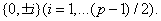Let f be a function from Rp to Z such that f (a) has the same notation in Z as a in Rp. Then for elements a∈Rp such that |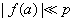, addition, subtraction and multiplication are the same as in Z. In other words, for such elements we do not feel the existence of p . Indeed, let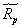be a subset of elements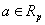such that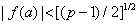. Then for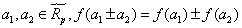and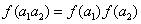which shows that f is a local isomorphism of some vicinities of zero in Rp and Z.

As explained in textbooks, both Rp and Z are cyclic groups with respect to addition. However, Rp has a higher symmetry because, in contrast to Z, Rp has a property which we call strong cyclicity: for any fixedany element of Rp distinct from a can be obtained from a by successively adding 1. In particular, by successively adding 1 to a "positive" element(i.e. such that f (a) > 0 we will get all such that the distance between the neighboring elements of Rp is unity. When p increases, the bigger and bigger part of Rp becomes the same as Z. Hence Z can be treated as a degenerate case of Rp in the formal limit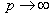because in this limit operations modulo p disappear and strong cyclicity is broken. Therefore, at the level of rings standard mathematics is a degenerate case of finite one when formally.

The transition from Rp to Z is similar to the procedure, which in group theory is called contraction. This notion is used when the Lie algebra of a group with a lower symmetry can be treated as a formal limit of the Lie algebra of a group with a higher symmetry when some parameter goes to zero or infinity. A well-known example in physics is that the Poincare group is the degenerate case of the de Sitter (dS) and anti-de Sitter (AdS) ones in the formal limit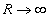or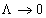where R is the radius of the world and Λ is the cosmological constant.

The above construction has a well-known historical analogy. For many years people believed that the Earth was flat and infinite, and only after a long period of time they realized that it was finite and curved. It is difficult to notice the curvature when we deal only with distances much less than the radius of the curvature. Analogously one might think that the set of numbers describing nature has a "curvature" defined by a very large number p but we do not notice it when we deal only with numbers much less than p .

One might argue that introducing a new fundamental constant p is not justified. However, history of physics tells us that more general theories arise when a parameter, which in the old theory was treated as infinitely small or infinitely large, becomes finite. For example, nonrelativistic physics is the degenerate case of relativistic one in the formal limit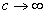(where c is the velocity of light), classical physics is the degenerate case of quantum one in the formal limit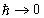and Poincare invariant theory is the degenerate case of dS or AdS invariant ones in the formal limitor. Therefore, it is natural to think that in quantum physics the quantity p should be not infinitely large but finite. A problem arises whether p is a constant or is different in various periods of time. In view of the problem of time in quantum theory, an extremely interesting scenario is that the world time is defined by p . In standard mathematics the next step is a transition from Z to the field of rational numbers Q. Historically the notions of infinitely small/large, continuity and standard division have arisen from a belief based on everyday experience that any macroscopic object can be divided into arbitrarily large number of arbitrarily small parts. However, the very existence of elementary particles indicates that those notions have only a limited meaning. Indeed, we can divide any macroscopic body by ten, million, etc. but when we reach the level of atoms and elementary particles the division operation loses its meaning and we cannot obtain arbitrarily small parts. Analogously, in any computer the number of bits can be only a positive integer and such notions as 1/2 bit, 1/3 bit etc. are meaningless. Those examples show that mathematics involving the set of all rational numbers has only a limited applicability and using standard mathematics in quantum physics and computer science is at least unnatural. Any description of macroscopic phenomena using continuity and differentiability can be only approximate. Water in the ocean can be described by differential equations of hydrodynamics but this is only an approximation since matter is discrete. In finite mathematics the ring Rp becomes the Galois field Fp if p is prime. For this transition no new elements are needed. While at the level of rings standard and finite mathematics can be treated as close to each other when p is large, at the level of fields they considerably differ each other. For example, 1/2 in Fp equals ( p +1) / 2, i.e. a very large number if p is large. However, this does not mean that mathematics modulo p cannot describe nature because spaces in quantum theory are projective. In Reference [1, 2] we have proposed an approach called GFQT where quantum theory is based on a Galois field with characteristic p . In our subsequent publications [3, 4] and references therein) this approach has been considerably developed. In particular, it has been shown that in the formal limitGFQT recovers predictions of standard continuous theory. Then the fact that standard mathematics describes many experiments with a high accuracy is a consequence of the fact that in real life the number p is very large.

In addition, GFQT gives a new look at many fundamental problems in physics [3,4]. We consider two examples which are strong indications that nature is described by finite mathematics.

A well-known fact of particle physics is that a particle and its antiparticle have equal masses. In standard particle physics this is explained as follows. By definition, according to Wigner's approach, a particle is called elementary if the space of its states is a space of an irreducible representation (IR) of the symmetry algebra or group. In Poincare and AdS theories there are two types of IRs such that the energy is either strictly positive or strictly negative. By definition, particles are described by positive energy IRs while antiparticles - by negative energy IRs (and after quantization the energy becomes positive for both particles and antiparticles). Then the fact that a particle and its antiparticle have equal masses follows from the requirement that their IRs should be combined into a local field satisfying a covariant equation (e.g. the Dirac equation). A question arises that if locality is only approximate then the masses of a particle and its antiparticle remain equal or can differ each other? This question is legitimate because, since local fields are described by non-unitary representations, their probabilistic interpretation is problematic.

Irreducible representations corresponding to particles are constructed from states where energy=mass. When representation operators act on such states the energy can only increase and we get the spectrum of energies in the range [mass,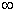). In mathematics such IRs are called IRs with the minimum weight. Analogously, in the case of antiparticles we start from states where energy=-mass. Then the energy can only decrease and we get IRs with the maximum weight where the spectrum of energies is in the range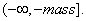Hence a particle and its antiparticle are de-scribed by different IRs and the equality of their masses is a consequence of additional requirements. However, in dS theory IRs have both, positive and negative energies. As shown in , the only possible interpretation of such IRs is that they describe a particle and its antiparticle simultaneously. Then even the notions of a particle and antiparticle can be only approximate. Since in this case a particle and its antiparticle belong to the same IR, their masses are automatically equal.

In GFQT we also start from states where energy=mass and gradually increase the energy by acting by representation operators on such states. However, in such a way we are moving not along a straight line but along the ring Rp in Figure1. The sooner or later we will arrive at the point where energy=-mass which shows that a finite field analog of an IR with the minimum weight is simultaneously a finite field analog of an IR with the maximum weight. Therefore in GFQT a particle and its antiparticle automatically belong to the same IR and have the same masses because the ring Rp is finite and has the property of strong cyclicity.

As discussed in , the notion when states with positive energies can be called particles and states with negative energies | antiparticles is valid only when p is rather large. In general, transitions particle ↔ antiparticle are not prohibited. As a consequence, conservation of electric charge and baryon and lepton quantum numbers can be only approximate. If at early stage of the World the value of p was much less than now then such transitions had much greater probabilities. This scenario gives a natural explanation of the problem known as "baryon asymmetry of the World" (that at present the number of particles in the World is much greater than the number of antiparticles).

Another striking example is that gravity can be treated not as an inter-action but simply as a manifestation of the fact that nature is described by a Galois field of characteristic p . In this approach the gravitational constant G is not a parameter taken from the outside (e.g. from the condition that theory should describe experiment) but a quantity which should be calculated. The actual calculation is problematic but reasonable qualitative arguments show  that G is proportional to 1/ln p . Therefore, gravity is a consequence of the finiteness of nature and disappears in the limit. A qualitative estimation based on additional assumptions gives that p is a huge number of the order of exp (1080).

As noted above, in quantum theory division has a limited applicability. This might be an indication that (as Metod Saniga pointed out), in the spirit of , the ultimate quantum theory will be based even on a finite ring and not a field.

The absolute majority of mathematicians and physicists think that standard mathematics is fundamental while finite one is inferior. Typical reasons are that standard mathematics contains more numbers than finite one and that the whole history of mankind has proven that standard mathematics describes reality with an unprecedented accuracy. However, as explained above, in the formal limitoperations modulo p disappear and strong cyclicity is broken. Therefore standard mathematics is a less symmetric degenerate case of finite one in the formal limit. This fact is obvious and probably it has been overlooked by mathematicians. We have also argued that finite mathematics is more pertinent for describing nature than standard one. An illusion of continuity arises because p is very large.

We now consider Problem 2 which has a long history . As shown by Russell and other mathematicians, the Cantor set theory contains several fundamental paradoxes. To avoid them several axiomatic set theories have been proposed and the most known of them is the ZFC theory developed by Zermelo and Fraenkel. The usual statements in set theory are that the consistency of ZFC cannot be proven within ZFC itself and the continuum hypothesis is independent of ZFC. Those statements have been questioned by Woodin  and the problem is open. Gὅdel's incompleteness theorems state that no system of axioms can ensure that all facts about natural numbers can be proven and the system of axioms in traditional mathematics cannot demonstrate its own consistency.

In constructive mathematics proposed by Brouwer there is no law of the excluded middle and it is required that any proof of existence be algorithmic. That is why constructive mathematics is treated such that, at least in principle, it can be implemented on a computer. Here "in principle" means that the number of steps might be not finite. With this meaning constructive mathematics, as well as traditional one assumes that one can operate with any desired amount of resources and it is theoretically possible to consider an idealized case when a computer can operate with any desirable number of bits.

The absolute majority of mathematicians prefer the traditional version. Physics is also based only on traditional mathematics. Hilbert was a strong opponent of constructive mathematics. He said: "No one shall expel us from the paradise that Cantor has created for us" and "Taking the principle of excluded middle from the mathematician would be the same, say, as proscribing the telescope to the astronomer or to the boxer the use of his fists".

Some well-known results of traditional mathematics are counterintuitive. For example, since the mapping tgx from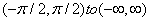is a bijection, those intervals have the same number of elements although the former is a part of the latter. Another example is Hilbert's Grand Hotel paradox. However, in traditional mathematics those examples are not treated as contradictory.

Let us look at mathematics from the point of view of philosophy of quantum theory according to which (in contrast to religion) there should be no statements accepted without proof and based on belief in their correctness (i.e. axioms). The theory should contain only those statements that can be verified, where by "verified" physicists mean an experiment involving only a finite number of steps.

Let us pose a problem of whether 10+20 equals 30. Then we should describe an experiment which should solve this problem. Any computer can operate only with a finite number of bits and can perform calculations only modulo some number p . Say p = 40, then the experiment will confirm that 10+20=30 while if p = 25 then we will get that 10+20=5. So the statements that 10+20=30 and even that 2.2=4 are ambiguous because they do not contain information on how they should be verified. We believe the following observation is very important: although standard mathematics is a part of our everyday life, people typically do not realize that standard mathematics is implicitly based on the assumption that one can have any desirable amount of resources. So standard mathematics (including traditional and constructive versions) is based on the implicit assumption that we can consider a formal limitand the correctness of the limit can be substantiated.

While Gὅdel's works on the incompleteness theorems are written in highly technical terms of mathematical logics, the fact that standard mathematics has foundational problems is clear from the philosophy of quantum theory. For instance, the first incompleteness theorem says that not all facts about natural numbers can be proven. However, from the philosophy of quantum theory this seems to be clear because if the number of numbers is not finite then we cannot verify that a + b = b + a for any a and b . The above discussion indicates that, from the point of view of philosophy of quantum theory, foundation of finite mathematics is natural while foundation of standard one encounters serious problems. However, since standard mathematics is a degenerate case of finite one, standard mathematics might be treated only as a technique which in many cases describes reality with a high accuracy while the fact that this mathematics has foundational problems does not have a fundamental role. The philosophy of Brouwer, Cantor, Fraenkel, Gὅdel, Hilbert, Kronecker, Russell, Zermelo and other great mathematicians working on foundation of standard mathematics was based on macroscopic experience in which the notions of in finitely small, infinitely large, continuity and standard division are natural. However, as noted above, those notions contradict the existence of elementary particles and are not natural in quantum theory.

#### Acknowledgements

I am very grateful to Sergey Dolgobrodov, Volodya Netchitailo and Teodor Shtilkind for numerous stimulating discussions and to the anonymous referee for important remark.

#### References

Select your language of interest to view the total content in your interested language

### Article Usage

• Total views: 16229
• [From(publication date):
April-2015 - Jan 22, 2020]
• Breakdown by view type
• HTML page views : 12455Can't read the image? click here to refresh